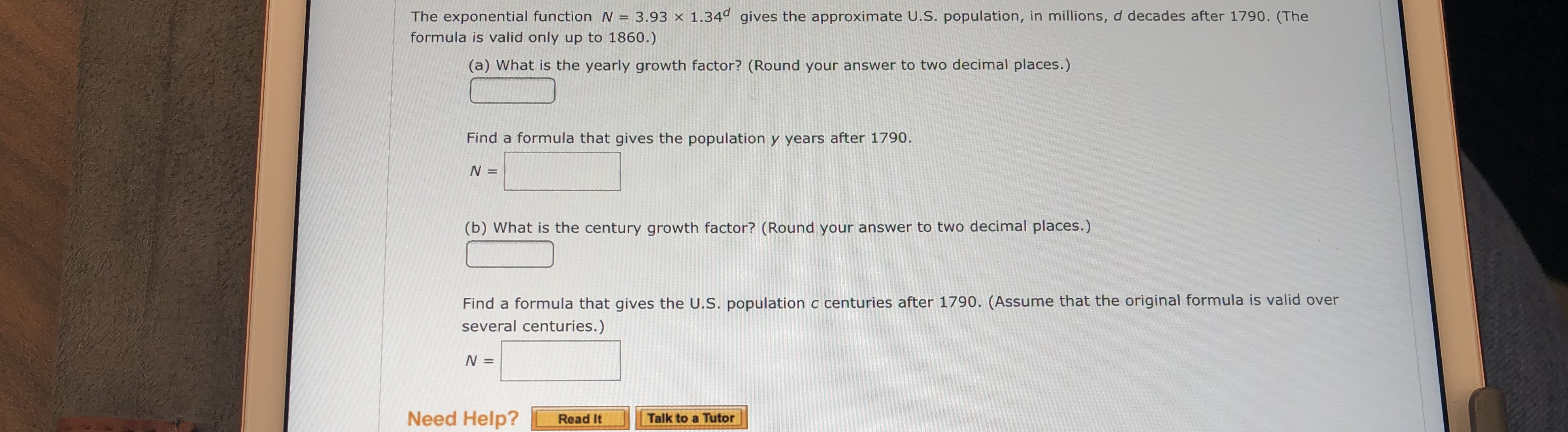# The exponential function N 3.93 x 1.34d gives the approximate U.S. population, in millions, d decades after 1790. (The formula is valid only up to 1860.) (a) What is the yearly growth factor? (Round your answer to two decimal places.) Find a formula that gives the population y years after 1790 N = (b) What is the century growth factor? (Round your answer to two decimal places.) Find a formula that gives the U.S. population c centuries after 1790. (Assume that the original formula is valid over several centuries.) N = Need Help? Read It Talk to a Tutor

Question

What are the blanks?help_outlineImage TranscriptioncloseThe exponential function N 3.93 x 1.34d gives the approximate U.S. population, in millions, d decades after 1790. (The formula is valid only up to 1860.) (a) What is the yearly growth factor? (Round your answer to two decimal places.) Find a formula that gives the population y years after 1790 N = (b) What is the century growth factor? (Round your answer to two decimal places.) Find a formula that gives the U.S. population c centuries after 1790. (Assume that the original formula is valid over several centuries.) N = Need Help? Read It Talk to a Tutor fullscreen

### Want to see this answer and more?

Experts are waiting 24/7 to provide step-by-step solutions in as fast as 30 minutes!*

*Response times may vary by subject and question complexity. Median response time is 34 minutes for paid subscribers and may be longer for promotional offers.
Tagged in
Math
Algebra

### Other